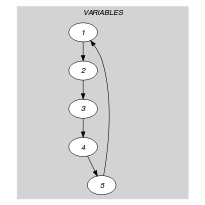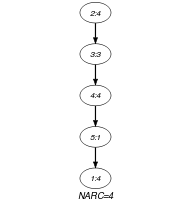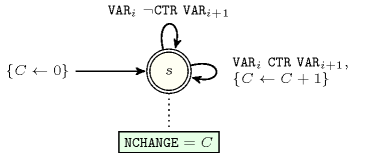## 5.68. circular_change

Origin

Derived from $\mathrm{𝚌𝚑𝚊𝚗𝚐𝚎}$.

Constraint

$\mathrm{𝚌𝚒𝚛𝚌𝚞𝚕𝚊𝚛}_\mathrm{𝚌𝚑𝚊𝚗𝚐𝚎}\left(\mathrm{𝙽𝙲𝙷𝙰𝙽𝙶𝙴},\mathrm{𝚅𝙰𝚁𝙸𝙰𝙱𝙻𝙴𝚂},\mathrm{𝙲𝚃𝚁}\right)$

Arguments
 $\mathrm{𝙽𝙲𝙷𝙰𝙽𝙶𝙴}$ $\mathrm{𝚍𝚟𝚊𝚛}$ $\mathrm{𝚅𝙰𝚁𝙸𝙰𝙱𝙻𝙴𝚂}$ $\mathrm{𝚌𝚘𝚕𝚕𝚎𝚌𝚝𝚒𝚘𝚗}\left(\mathrm{𝚟𝚊𝚛}-\mathrm{𝚍𝚟𝚊𝚛}\right)$ $\mathrm{𝙲𝚃𝚁}$ $\mathrm{𝚊𝚝𝚘𝚖}$
Restrictions
 $\mathrm{𝙽𝙲𝙷𝙰𝙽𝙶𝙴}\ge 0$ $\mathrm{𝙽𝙲𝙷𝙰𝙽𝙶𝙴}\le |\mathrm{𝚅𝙰𝚁𝙸𝙰𝙱𝙻𝙴𝚂}|$ $\mathrm{𝚛𝚎𝚚𝚞𝚒𝚛𝚎𝚍}$$\left(\mathrm{𝚅𝙰𝚁𝙸𝙰𝙱𝙻𝙴𝚂},\mathrm{𝚟𝚊𝚛}\right)$ $\mathrm{𝙲𝚃𝚁}\in \left[=,\ne ,<,\ge ,>,\le \right]$
Purpose

$\mathrm{𝙽𝙲𝙷𝙰𝙽𝙶𝙴}$ is the number of times that $\mathrm{𝙲𝚃𝚁}$ holds on consecutive variables of the collection $\mathrm{𝚅𝙰𝚁𝙸𝙰𝙱𝙻𝙴𝚂}$. The last and the first variables of the collection $\mathrm{𝚅𝙰𝚁𝙸𝙰𝙱𝙻𝙴𝚂}$ are also considered to be consecutive.

Example
$\left(4,〈4,4,3,4,1〉,\ne \right)$

In the example the changes within the $\mathrm{𝚅𝙰𝚁𝙸𝙰𝙱𝙻𝙴𝚂}=〈4,4,3,4,1〉$ collection are located between values 4 and 3, 3 and 4, 4 and 1, and 1 and 4 (i.e., since the third argument $\mathrm{𝙲𝚃𝚁}$ of the $\mathrm{𝚌𝚒𝚛𝚌𝚞𝚕𝚊𝚛}_\mathrm{𝚌𝚑𝚊𝚗𝚐𝚎}$ constraint is set to $\ne$, we count one change for each disequality constraint between two consecutive variables that holds). Consequently, the corresponding $\mathrm{𝚌𝚒𝚛𝚌𝚞𝚕𝚊𝚛}_\mathrm{𝚌𝚑𝚊𝚗𝚐𝚎}$ constraint holds since its first argument $\mathrm{𝙽𝙲𝙷𝙰𝙽𝙶𝙴}$ is fixed to 4.

Typical
 $\mathrm{𝙽𝙲𝙷𝙰𝙽𝙶𝙴}>0$ $|\mathrm{𝚅𝙰𝚁𝙸𝙰𝙱𝙻𝙴𝚂}|>1$ $\mathrm{𝚛𝚊𝚗𝚐𝚎}$$\left(\mathrm{𝚅𝙰𝚁𝙸𝙰𝙱𝙻𝙴𝚂}.\mathrm{𝚟𝚊𝚛}\right)>1$ $\mathrm{𝙲𝚃𝚁}\in \left[\ne \right]$
Symmetries
• Items of $\mathrm{𝚅𝙰𝚁𝙸𝙰𝙱𝙻𝙴𝚂}$ can be shifted.

• One and the same constant can be added to the $\mathrm{𝚟𝚊𝚛}$ attribute of all items of $\mathrm{𝚅𝙰𝚁𝙸𝙰𝙱𝙻𝙴𝚂}$.

Arg. properties

Functional dependency: $\mathrm{𝙽𝙲𝙷𝙰𝙽𝙶𝙴}$ determined by $\mathrm{𝚅𝙰𝚁𝙸𝙰𝙱𝙻𝙴𝚂}$ and $\mathrm{𝙲𝚃𝚁}$.

Keywords
Arc input(s)

$\mathrm{𝚅𝙰𝚁𝙸𝙰𝙱𝙻𝙴𝚂}$

Arc generator
$\mathrm{𝐶𝐼𝑅𝐶𝑈𝐼𝑇}$$↦\mathrm{𝚌𝚘𝚕𝚕𝚎𝚌𝚝𝚒𝚘𝚗}\left(\mathrm{𝚟𝚊𝚛𝚒𝚊𝚋𝚕𝚎𝚜}\mathtt{1},\mathrm{𝚟𝚊𝚛𝚒𝚊𝚋𝚕𝚎𝚜}\mathtt{2}\right)$

Arc arity
Arc constraint(s)
$\mathrm{𝚟𝚊𝚛𝚒𝚊𝚋𝚕𝚎𝚜}\mathtt{1}.\mathrm{𝚟𝚊𝚛}\mathrm{𝙲𝚃𝚁}\mathrm{𝚟𝚊𝚛𝚒𝚊𝚋𝚕𝚎𝚜}\mathtt{2}.\mathrm{𝚟𝚊𝚛}$
Graph property(ies)
$\mathrm{𝐍𝐀𝐑𝐂}$$=\mathrm{𝙽𝙲𝙷𝙰𝙽𝙶𝙴}$

Graph model

Since we are also interested in the constraint that links the last and the first variable we use the arc generator $\mathrm{𝐶𝐼𝑅𝐶𝑈𝐼𝑇}$ to produce the arcs of the initial graph.

Parts (A) and (B) of Figure 5.68.1 respectively show the initial and final graph associated with the Example slot. Since we use the $\mathrm{𝐍𝐀𝐑𝐂}$ graph property, the arcs of the final graph are stressed in bold.

##### Figure 5.68.1. Initial and final graph of the $\mathrm{𝚌𝚒𝚛𝚌𝚞𝚕𝚊𝚛}_\mathrm{𝚌𝚑𝚊𝚗𝚐𝚎}$ constraint(a) (b)
Automaton

Figure 5.68.2 depicts the automaton associated with the $\mathrm{𝚌𝚒𝚛𝚌𝚞𝚕𝚊𝚛}_\mathrm{𝚌𝚑𝚊𝚗𝚐𝚎}$ constraint. To each pair of consecutive variables $\left({\mathrm{𝚅𝙰𝚁}}_{i},{\mathrm{𝚅𝙰𝚁}}_{\left(i\mathrm{mod}|\mathrm{𝚅𝙰𝚁𝙸𝙰𝙱𝙻𝙴𝚂}|\right)+1}\right)$ of the collection $\mathrm{𝚅𝙰𝚁𝙸𝙰𝙱𝙻𝙴𝚂}$ corresponds a 0-1 signature variable ${S}_{i}$. The following signature constraint links ${\mathrm{𝚅𝙰𝚁}}_{i}$, ${\mathrm{𝚅𝙰𝚁}}_{\left(i\mathrm{mod}|\mathrm{𝚅𝙰𝚁𝙸𝙰𝙱𝙻𝙴𝚂}|\right)+1}$ and ${S}_{i}$: ${\mathrm{𝚅𝙰𝚁}}_{i}\mathrm{𝙲𝚃𝚁}{\mathrm{𝚅𝙰𝚁}}_{\left(i\mathrm{mod}|\mathrm{𝚅𝙰𝚁𝙸𝙰𝙱𝙻𝙴𝚂}|\right)+1}⇔{S}_{i}$.

##### Figure 5.68.2. Automaton of the $\mathrm{𝚌𝚒𝚛𝚌𝚞𝚕𝚊𝚛}_\mathrm{𝚌𝚑𝚊𝚗𝚐𝚎}$ constraint##### Figure 5.68.3. Hypergraph of the reformulation corresponding to the automaton of the $\mathrm{𝚌𝚒𝚛𝚌𝚞𝚕𝚊𝚛}_\mathrm{𝚌𝚑𝚊𝚗𝚐𝚎}$ constraint